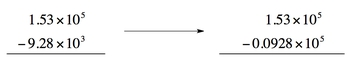## General Chemistry

Learn the toughest concepts covered in Chemistry with step-by-step video tutorials and practice problems by world-class tutors

5. BONUS: Mathematical Operations and Functions

Addition and subtraction of values in scientific notation.

Whenever we add or subtract values in scientific notation we must make sure the exponents are the same value.

1
concept1m
Play a video:
So here, when you add or subtract values and scientific notation, they must have the same exponents. So the coefficients will add or subtract, depending on what operation is going on. But the exponents remained constant. So here we have eight times, 10 to the X minus B times 10 to the X. Again, both of them must have the same exponents to the same power in order to add or subtract with one another. So here this will just become a minus bi times 10 to the X and then if we're adding them, would be a plus B again times 10 to the X. Now there will come situations where the exponents do not match. What do we do in those situations we're going to save? The exponents are not the same that we transform the smaller value so that they dio. So let's say that you had one value that was times 10 to the eight, and the other one was times 10 to the five. You'd have to increase the 10 to the 5 to 10 38 so that both of them would have the same exponents. Then we could either subtract or add them Thio together. Now, it says, Remember when adding or subtracting values that the final answer must have the least number off decimal places. So when it comes to adding or detracting, we want least number of decimal places in our coefficient. But we're multiplying or dividing its least number of significant figures. Now that we've learned the basics in terms of this in these operations, let's take a look at example, one. You can attempt to try to do it on your own. If you get stuck, come back and take a look at my video and see how I approach this very question.

If the exponents are not the same then we transform the smaller value so that they do.2
concept

## Addition and Subtraction Calculations 12m
Play a video:
using the method discussed above. Determine the answer. The following question. So here it's 8.17 times 10 to the eight plus 1.25 times 10 to the nine. 10 to the nine is the larger power. So that's the larger value. So that would mean that we need to convert the smaller value to match that same exponents. So here we have 10 to the eight. So we need to increase it by one. Now, if we're trying to increase the exponents by one, So we're gonna increase by one. That means that we're gonna have to decrease your coefficient by one decimal place. So remember, there is ah, reciprocal or opposite relationship between your coefficient and your exponents. Whatever happens toe one, the opposite happens to the other. So we're gonna need to make this numbers this coefficient value smaller. That my power of eight can increase to become the power of nine. So I'm gonna take this decimal. I'm gonna move it over one so that we go from 8. point And by making that smaller, this just became larger. So plus 1.25 times to the nine. Now that both of them have the same exact exponents, I can finally add them together. The exponents stays constant and all I'm doing now is I'm adding 0.817 plus 1. So when I add those two together gives me 2. times 10 to the ninth. But remember, when we're adding or subtracting coefficients, we want the least number of decimal places. So for the first value, we have three decimal places. Remember, decimal places are the numbers to the right of the decimal point. This has three decimal places. This year has two decimal places. So my answer at the end must have two decimal places total. So we're gonna have to round this 22.7 There will be times 10 to the nine. So that would be my final answer here. Following the rules that we observed up above
3
concept

## Addition and Subtraction Calculations 21m
Play a video: